# Difference between revisions of "Banked turn at constant speed and constant height with no slipping"

This article discusses a scenario/arrangement whose statics/dynamics/kinematics can be understood using the ideas of classical mechanics.
View other mechanics scenarios

This article discusses a scenario where a vehicle with wheels is making a banked turn with a banking angle of$\theta$ without slipping and without any change in its altitude (i.e. it is not trying to go uphill or downhill).

## Basic components of force diagram

### The three candidate forces

Force (letter) Nature of force Condition for existence Magnitude Direction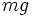$mg$ gravitational force unconditional$mg$ where$m$ is the mass and$g$ is the acceleration due to gravity vertically downward, hence an angle$\theta$ with the normal to the incline and an angle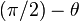$(\pi/2) - \theta$ with the incline$N$ normal force unconditional adjusts so that there is no net acceleration perpendicular to the plane of the incline outward normal to the incline$f_s$ static friction no sliding adjusts so that there is no net acceleration along the incline it is along the incline either up or down. Note that if the vehicle's speed were changing, then the friction force would have a component up the incline and a component opposite the direction of motion of the vehicle.

## Equations

AMBIGUOUS DIRECTION: The direction of the static friction force$f_s$ has a sense ambiguity, i.e., we are not sure whether it acts up the incline or down the incline. In order to avoid the ambiguity, we will assume a downward (inward) positive convention but allow$f_s$ to have both positive and negative measurement.

We choose to take components in the following three dimensions: the vertical direction, the horizontal direction that is perpendicular to the direction of motion of the vehicle, and the horizontal direction of motion of the vehicle.

It turns out that all the forces are in the plane spanned by the first two dimensions.

The vehicle has no acceleration in the vertical direction, so Newton's first law of motion gives us that:$\! mg = N \cos \theta \qquad (1.1)$

The acceleration in the horizontal direction perpendicular to the motion of the vehicle is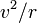$v^2/r$ where$v$ is the speed and$r$ is the radius of curvature at the point of the curve obtained by taking a cross section at that vertical height. If the cross section is circular, then$r$ is the radius of the circle.

We thus get, by Newton's second law of motion, that: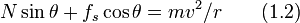$\! N \sin \theta + f_s \cos \theta = mv^2/r \qquad (1.2)$

We also have:$\! |f_s| \le \mu_sN \qquad (1.3)$

### Relation between limiting coefficient of static friction, radius of curvature, banking angle, and speed of turn

Plugging (1.1) into (1.2), we get:$\! f_s = m\frac{v^2/r - g \tan \theta}{\cos \theta} \qquad (2.1)$

Plugging (1.1) into (1.3), we have: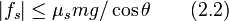$\! |f_s| \le \mu_smg/\cos \theta \qquad (2.2)$

Combining (2.1) and (2.2) we get:

$v^2}{r$

Rearranging, we get: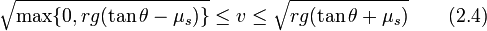$\sqrt{\max \{ 0, rg (\tan \theta - \mu_s) \} } \le v \le \sqrt{rg(\tan \theta + \mu_s)} \qquad(2.4)$

In particular, in the frictionless case we would get$v = \sqrt{rg \tan \theta}$. Note that the presence of static friction provides some leeway and allows a range of possible speeds rather than a unique single speed that works.

### Two cases

We consider two cases:

•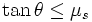$\tan \theta \le \mu_s$, so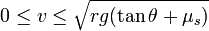$0 \le v \le \sqrt{rg(\tan \theta + \mu_s)}$: In other words, the banking angle is less than or equal to the angle of friction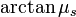$\arctan \mu_s$. In this case, the vehicle could be parked at the angle without downward slipping. There is thus no lower limit to the speed at which it can negotiate the turn. However, there is an upper limit of$\sqrt{rg(\tan \theta + \mu_s)}$.
•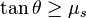$\tan \theta \ge \mu_s$, so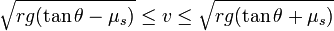$\sqrt{rg(\tan \theta - \mu_s)} \le v \le \sqrt{rg(\tan \theta + \mu_s)}$: In this case, there is both a minimum and a maximum speed needed to negotiate the turn at constant speed. The reason for a minimum speed is that if the vehicle is too slow, then it will slip downward because of gravity. To counteract that, a sufficiently high normal force must be applied which in turn causes a significant centripetal force which in turn causes a high centripetal acceleration, which is consistent only with a certain minimum speed because of math>a = v^2/r[/itex].

## Confusion with sliding motion along an inclined plane

The basic forces operating in the case of a banked turn are similar to the basic forces operating for sliding motion along an inclined plane, yet the analyses for these two situations are fairly different. We now examine how and why the analyses differ.

### Direction of acceleration and no acceleration

In the case of sliding motion along an inclined plane, the block could potentially move up or down the incline. Thus, the direction of acceleration is potentially parallel to the incline in the up-down direction. There is no acceleration in the direction normal to the incline.

In the case of a banked turn, the body is moving along a curve at constant speed, hence its direction of acceleration is determined by the normal direction to the curve of motion. The curve of motion is (by assumption) at a constant height so the direction of acceleration is horizontal, perpendicular to the direction of motion (in our diagrammatic depiction, the direction of motion is perpendicular to the plane being depicted). There is no acceleration in the purely vertical direction.

### Choice of axes to take components

Once we have the force equations as vector equations, it is possible to take coordinates (i.e. take components of forces) with any system of mutually perpendicular axes, and then set up and solve the equations. In most cases, though, there is a convenient choice of axes that makes the equation setup and solving as easy as possible. Usually, this is done as follows: one axis is the direction of possible acceleration, the second axis is an orthogonal direction where the forces have nonzero components but there is no net acceleration, and the third axis is the one orthogonal to both of these.

Based on our discussion above, we see that for the case of sliding motion along an inclined plane, the best choice of axes is along the plane (up/down) (the direction of possible acceleration), perpendicular to the plane (the direction of the normal force and a component of the gravitational force), and along the plane (horizontal) (no forces, no acceleration).

In the case of a banked turn at a constant speed and constant height, on the other hand, the best choice of axes is horizontal, perpendicular to direct of motion (direction of acceleration -- centripetal), vertical (direction of gravitational force, other forces), and horizontal, along motion (no force or acceleration).

### Comparison of specific equations

Contrast the equation for sliding motion along an inclined plane:

Failed to parse (unknown function "\mg"): \! N = \mg\cos \theta

against the equation for banked turn with no slippage: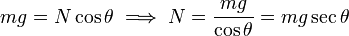$\! mg = N \cos \theta \implies N = \frac{mg}{\cos \theta} = mg \sec \theta$

In the case of sliding motion along an inclined plane, the normal force is smaller in magnitude than the gravitational force; in fact, it is$\cos \theta$ times the gravitational force and thus successfully counteracts the component of gravitational force in the direction normal to the incline. The component along the incline, namely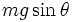$mg\sin \theta$, is not canceled or affected by the normal force, though it could be partly or completely countered by the friction force. In other words, the vehicle is pressed against the surface less strongly than its weight.

In the case of a banked turn the normal force is greater in magnitude than the gravitational force, so that it completely cancels the gravitational force in the pure vertical direction, and the remaining component serves the role of centripetal acceleration. In other words, the vehicle is pressed against the surface more strongly than its weight.

### How the stationary case of sliding motion along an inclined plane is a special case of this

The stationary case of sliding motion along an inclined plane can be viewed as a special case of this where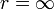$r = \infty$, i.e., there is no turn. We see that in this case, the only situation where the vehicle can maintain a constant height is if$\tan \theta \le \mu_s$.